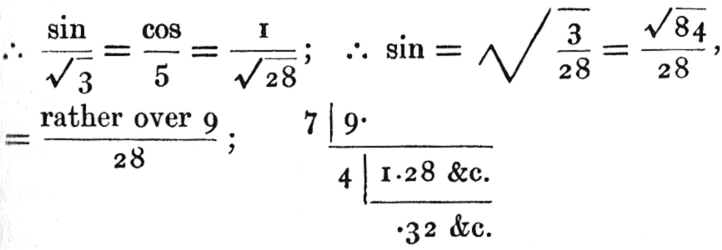#Pillow-Problems: Problem #70

Math Lair Home > Source Material > Pillow-Problems > Problem #70

## Problem:

70. (27, 105)

Let an equilateral and equilangular Tetrahedron be placed with one facet in front: and suppose a series of triangles, equal to that facet, constructed in the Plane containing that facet, and having a base common with it; and that they are all wrapped round the Tetrahedron as far as they will go. Find (1) the locus of their vertices; (2) the situation of the vertex of the one whose left-hand base-angle is 15°; (3) the left-hand base-angle of the one which (wrapped round towards the right) covers portions of all four facets of the Tetrahedron, and whose vertex coincides with its vertex; (4) the left-hand base-angle of the one which (similarly treated) occupies all four facets, and then the front and right-hand facet of the second time, and whose vertex coincides with the distal vertex of the base of the Tetrahedron.

70. (17, 105)

(1) Down the back-edge; up again; and so on. (2) about .7 of the way down the back-edge. (3) about 18.65°. (4) About 14.53°.

## Solution:

70. (17, 27)

Before folding the Plane containing the Triangles, the locus of their vertices is evidently a Line parallel to their common base. Hence, if the base of the Tetrahedron = 1, we may imagine a slip of paper, whose width is
 (√3)/ (2)
, attached to the front facet of the Tetrahedron, and wrapped round towards the right; and the upper edge of this slip will evidently be the locus of the vertices. This slip may be conveniently regarded as divided into equilateral Triangles, placed base-downwards and base-upwards alternately, and it is evident that these Triangles will successively cover the facets of the Tetrahedron, in the order ‘front, right side, base, left side, front, &c.’; and its upper edge, made up of the bases of the inverted constituent Triangles, will evidently run as follows. Calling the successive Triangles, after the first (which occupies the front facet of the Tetrahedron), ‘α’ (base-up), ‘β’ (base-down), ‘γ’ (base-up), ‘δ’ (base-down), ‘ε’ (base-up), and so on, the locus consists of the bases of α γ &c. Now ‘α’ will occupy the right facet, its base coinciding with the back-edge of the Tetrahedron; ‘β’ will occupy the base of the Tetrahedron, its base coinciding with the front-edge; ‘γ’ will occupy the left facet, its base coinciding with the back-edge; and so on. Hence the locus runs down the back-edge; up again; and so on. Which answers Question (1).

Q.E.F.

We may therefore, in answering the other three questions, consider the slip before it is folded, and calculate the positions of the vertices along its upper edge: and the problems thus become ‘plane’ ones.

(2) Gives us a right-angled Triangle, whose left-hand base angle is 15°, and whose altitude is
 (√3)/ (2)
We must calculate its base, and then, deducting half the base of the initial Triangle (i.e. deducting ½), we shall get the distance, measured along the upper edge of the slip, from the vertex of the initial Triangle to the vertex off the given Triangle; and from that we can calculate how many times we must go down and up the back-edge to reach it. Call the base of this right-angled Triangle ‘x’. Then
 (√3)/ (2)
÷x = tan 15°.

Now call tan 15° ‘t’; then
 (2t)/ (1 − t²)
= tan 30° =
 (1)/ (√3)
;

∴ 1 − t² = 2√3 · t; t² + 2√3 · that − 1 = 0;

t =
 (−2√3 ± 4)/ (2)
= (rejecting negative value) 2 − √3.

x =
 (√3)/ (2(2 − √3))

 (√3)/ (2)
(2 + √3) = √3 +
 (3)/ (2)
.

Deducting ½, we get (√3 + 1) as the required distance.

Now √3 = 1.7 &c.; ∴ distance = 2.7 &c.

Hence we must go down back-edge, up again, and then about 7 down again. This answers question (2).

(3) We need to go down the back-edge, and up again; i.e. we must use the upward bases of ‘α’ and ‘γ’. Hence the base of the required right-angled Triangle is 2½. Hence the required left-hand base-angle is

tan−1 (
 (√3)/ (2)
÷
 (5)/ (2)
); i.e. tan−1
 (√3)/ (5)
.

Hence, for the required base-angle, we have
 (sin)/ (cos)
=
 (√3)/ (5)
;Now (by mem. tech.) sin−1 .3 = 17.45 &c°. sin−1 .4 = 23.57 &c°.
and the required angle is about
 (1)/ (5)
of the way from one to the other. But the difference is almost exactly 6°. Hence we must add, to the lesser, about 1
 (1)/ (5)
degrees, or 1.20°. And the total will be about 18.65°.

(4) Here the right-angled Triangle, has, for its base, 3½.

∴ the required base-angle has, for its tangent,

(
 (√3)/ (2)
÷
 (7)/ (2)
); i.e.
 (√3)/ (7)
;

 (sin)/ (√3)
=
 (cos)/ (7)
=
 (1)/ (√52)
; ∴ sin = √
 (3)/ (52)
= nearly √
 (1)/ (17)
, = nearly
 (√17)/ (17)
. Now √17 = 4.12 &c. ∴ sin = .24 &c.

Now sin−1 .2 = 11.53 &c°.; and we must go about half−way to the next angle, viz. 17.45 &c°. The difference is about 6°; ∴ we must add about 3°. Hence the answer is about 14.53°.